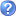• Significant Figures Java Applet
Requires correct identification of number of Significant Figures in numbers and (addition) equations. Here is a little game with significant figures. There are 12 different numbers for which you have to determine the number of significant figures. Try to keep the number of wrong tries to a minimum!
• General Chemistry Online: Companion Notes: Measurement
Measurement Learning Objectives A checklist of concepts to learn and skills to master in this section. Lecture Slides Lecture Notes Links Internet sites and paper references for further exploration. Frequently Asked Questions Find an answer, or ask a question. Glossary Terms and definitions from the glossary are marked with an asterisk (*). Quiz: Significant Figures A general quiz on significant figures. /chem/senese/101/measurement/sigfig-quiz.shtml (01/15/00) Tutorial: Arithmetic with units Focuses on units during addition, subtraction, multiplication, and division of measurements. This tutorial is an essential preliminary for unit conversion; it does not cover the arithmetic of significant figures. /chem/senese/101/measurement/index.
• Math Skills - Significant Figures
In any measurement, the number of significant figures is critical. The number of significant figures is the number of digits believed to be correct by the person doing the measuring. It includes one estimated digit. So, does the concept of significant figures deal with precision or accuracy? I'll answer this question after you peruse the next example. Let's look at an example where significant figures is important: measuring volume in the laboratory. This can be done in many ways: using a beaker with volumes marked on the side, a graduated cylinder, or a buret. Which glassware would give you the most precise volume measurement? Let's figure out the volume for each one a
• Measurement - a list of links to lots of stuff measurement
This particular anchor is "Metric System" Metric Prefixes; Metric Symbols; Metric Number Values; Metric Values in Words
• Measurement -- Volume and Capacity: Introduction
This webquest is set up to review the mathematical concepts of measurement and conversion with both the customary and the metric systems. It is my goal for these students to connect what they know about measurements to relationships they must use in science. My students should finish this quest with a better understanding of the interconnectedness of volume and capacity. Introduction Each student will have received 70 % or better on the pretest before beginning this quest. Those who need to will be getting some extra review before starting the quest.    As you will be using measurements in both math and science with different processes, it is important that you  have mastered skills in the measurement area.
• Measures
Metric System
• Range of cell sizes
by 2 users
• Units of Measure WebQuest: Introduction
Comparing the Metric and U.S. systems of measurement. Introduction Introduction: Pretend that our school has decided to secede from the United States and become its own nation, “The United People of Knowledge.” There are many tasks ahead of your new country: electing a leader, writing a new set of laws, choosing a currency, creating a new flag, etc. One very important task to be preformed is to establish a standard set of Weights and Measures.
• WebQuest: Our Country's Dominance in Question: created with Zunal WebQuest Maker 1
This WebQuest was designed to allow students to become more familiar with the metric system of measurement and to realize that a political battle is being waged over the measuring system we use. Depending on the number of students in your classroom and the amount of groups you like to have, you can either add temperature to choose from, or just allow groups to double up on selections. Also, you can have two groups select the same measurement and take opposing sides, one for the metric system and one against, so the students can have their own debate on the subject. I would recommend the students giving their own opinion, especially if it differs from the position they defended.